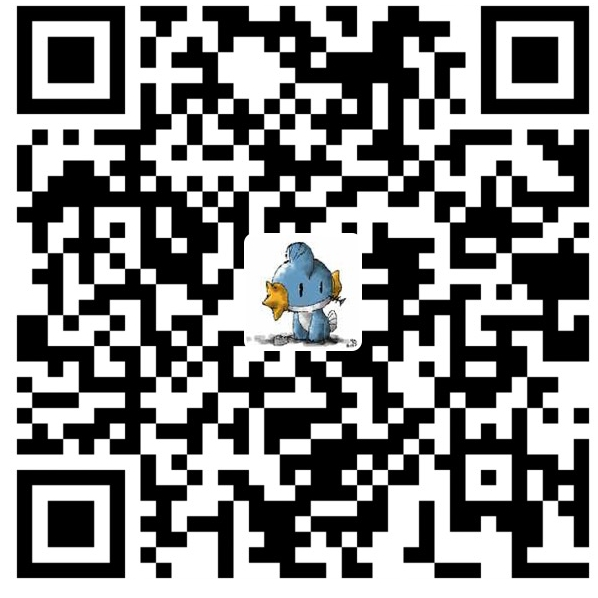# VBS字符串与UTF-16（Unicode）

VBS字符串的内部实现》中谈到VBS字符串内部是以wchar_t来实现的，而MSDN在《Unicode (Windows)》写道：

Unicode-enabled functions are described in Conventions for Function Prototypes. These functions use UTF-16 (wide character) encoding, which is the most common encoding of Unicode and the one used for native Unicode encoding on Windows operating systems.

VBS内部使用的是支持Unicode的Windows API函数，所以VBS字符串应该是支持UTF-16的。

```'Author: Demon
'Website: http://demon.tw
'Date: 2012/5/6
s = "𪚥"
WScript.Echo s
WScript.Echo Len(s)
For i = 1 To Len(s)
c = Mid(s, i, 1)
WScript.Echo Hex(AscW(c))
Next```

```'Author: Demon
'Website: http://demon.tw
'Date: 2012/5/6
s = "𪚥"
WScript.Echo ToCodePoint(s), Hex(ToCodePoint(s))

'return code point of a character
Function ToCodePoint(s)
Dim uni, uni2
uni = CLng("&H" & Hex(AscW(s)))
If (uni >= &HD800&) And (uni <= &HDBFF&) Then
uni2 = Mid(s, 2, 1)
If uni2 = "" Then
Err.Raise 8732, "ToCodePoint", _
"Invalid  surrogate pair"
End If
uni2 = CLng("&H" & Hex(AscW(uni2)))
uni = &H10000 + (((uni - &HD800&) * 2 ^ 10) Or (uni2 - &HDC00&))
End If
ToCodePoint = uni
End Function```

```'Author: Demon
'Website: http://demon.tw
'Date: 2012/5/6
WScript.Echo FromCodePoint(&H2A6A5)

'return a character from code point
Function FromCodePoint(n)
Dim s1, s2
If n < 0 Or n > &H10FFFF Then
Err.Raise 8732, "FromCodePoint", _
"Code point must be in 0-0x10FFFF"
ElseIf n < &H10000 Then
FromCodePoint = ChrW(n)
Else
n = n - &H10000
s1 = &HD800 Or ((n \ 2 ^ 10) And &H3FF)
s2 = &HDC00 Or (n And &H3FF)
FromCodePoint = ChrW(s1) & ChrW(s2)
End If
End Function```微信赞赏支付宝赞赏